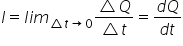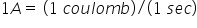# Current

The study of electric charges in motion is called current electricity.

Electric current :- the flow of electric charges through a conductor constitute electric current. For steady flow of charge, I=q/t

If the rate of flow of charge varies with time, thenSI unit of current is ampere.

If one coulomb of charge crosses an area in one sec., then current through that area is one ampere.Conventional current:  the direction of motion of positive charges is taken as the direction of conventional current.

Electronic current:-  the direction of flow of electrons gives the direction of electronic current.

Electric current is a scalar quantity :- the reason is that law of ordinary algebra are used to add electric current and laws of vector addition do not apply to the direction of electric current.

By maintaining the potential difference between the two ends of the conductor, current is kept contiuous inside a conductor.

Related Keywords